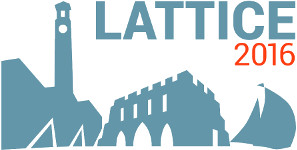#The 34th International Symposium on Lattice Field Theory (Lattice 2016)

Jul 24 – 30, 2016
Highfield Campus, University of Southampton
Europe/London timezone

## Form factors from moments of correlation functions

Jul 28, 2016, 2:40 PM
20m
Building B2a Room 2077 (Highfield Campus, University of Southampton)

### Speaker

Dr Chia Cheng Chang (LBNL)

### Description

Momentum-space derivatives of matrix elements can be related to their coordinate-space moments through the Fourier transform. We derive these expressions as a function of momentum transfer $Q^2$ for asymptotic in/out states consisting of a single hadron. We calculate corrections to the finite volume moments by studying the spatial dependence of the lattice correlation functions. This method permits the computation of not only the values of matrix elements at momenta accessible on the lattice, but also the momentum-space derivatives, providing *a priori* information about the $Q^2$ dependence of form factors. As a specific application we use the method, at a single lattice spacing and with unphysically heavy quarks, to directly obtain the slope of the isovector form factor at various $Q^2$, whence the isovector charge radius. The method has potential application in the calculation of any hadronic matrix element with momentum transfer, including those relevant to hadronic weak decays.

### Primary authors

Dr Chia Cheng Chang (LBNL) Dr Chris Bouchard (William &amp; Mary) Prof. David Richards (JLAB) Prof. Kostas Orginos (College of William and Mary/JLAB)

 Slides